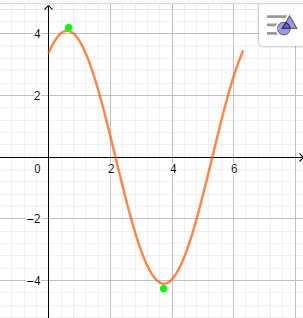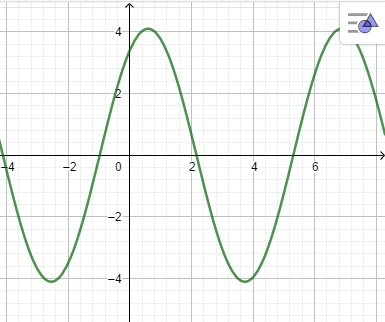# Given the function f( x ) = 2.3 \sin ( x ) + 3.4 \cos ( x ) . Find the maximum and minimum...

## Question:

Given the function {eq}f( x ) = 2.3 \sin ( x ) + 3.4 \cos ( x ) {/eq}. Find the maximum and minimum value of this function?

## Finding Maximum Value

With the first derivative of a function with one independent variable, we can find its maximum and minimum values, because the first derivative is the tangent line's slope.

The function has maximum and minimum values at the first derivative zero.

{eq}\displaystyle \ f'(x) \; = \; 2.3\,\cos \left( x \right) - 3.4\,\sin \left( x \right) \\ {/eq}

Then {eq}\displaystyle 0= 2.3\,\cos \left( x \right) - 3.4\,\sin \left( x \right) \; \Leftrightarrow \; x=0.5947592575 \; \text{ & } \; x= 3.736351911 \\ \; \text{ or } \; x=0.5947592575 +\pi \; \text{ & } \; x= 3.736351911+\pi \\ \; \text{ or } \; x=0.5947592575 +2\pi \; \text{ & } \; x= 3.736351911+2\pi \\ \; \text{ or } \; x=0.5947592575 +\pi \mathbb{N} \; \text{ & } \; x= 3.736351911+\pi \mathbb{N} \\ {/eq}

Remember, trigonometric functions has repetitive behavior. Then, if we choose a closed interval we can identify maximum and minimum values; we choose {eq}\displaystyle x \; \in [0,2\pi] {/eq}

Increasing and decreasing intervals:

{eq}\begin{array}{c} \; \text{Interval} \; & { 0 < x < 0.594759258 } & { 0.594759258 < x < 3.736351911 } & { 3.736351911 < x < 2\pi } \\ \hline Test \space{} value & { x = 0.5 } & { x = 2 } & { x = 6 } \\ Test & { f'( 0.5 ) = + > 0 } & { f'( 2 ) = - < 0 } & { f'( 6 ) = + > 0 } \\ Conclusion & { increasing } & { decreasing } & { increasing } \\ \end{array} \\ \therefore \boxed { \text{ decreasing's interval(s)} \; \ ( 0.594759258 , 3.736351911 )} \\ \therefore \boxed { \text{ increasing's interval(s)} \; \ ( 0 , 0.594759258) \cup ( 3.736351911 , 2\pi )} \\ {/eq}

Therefore, the function has maximum value at {eq}x=0.594759258 {/eq} and it is {eq}\displaystyle f(0.594759258)= 4.104875151 \\ {/eq}

and, the function has minimum value at

{eq}x=3.736351911 {/eq}

And, it is

{eq}\displaystyle f(3.736351911)=- 4.104875150 \\ {/eq}